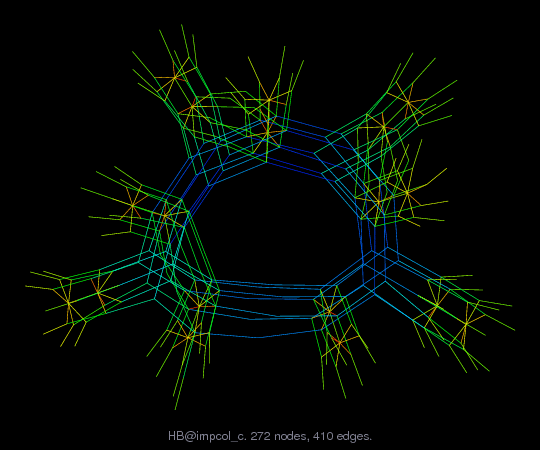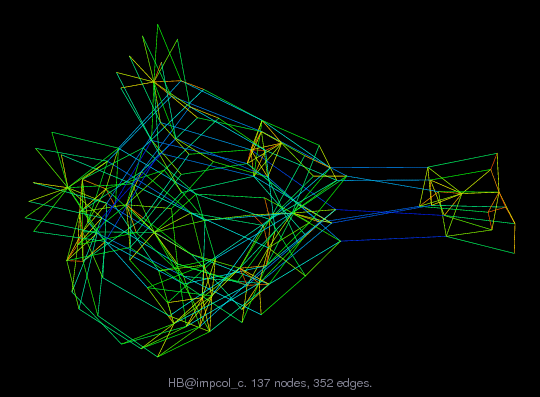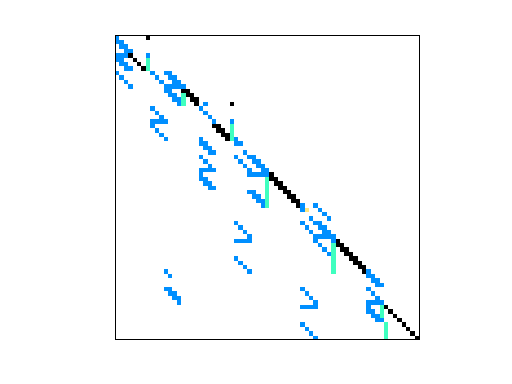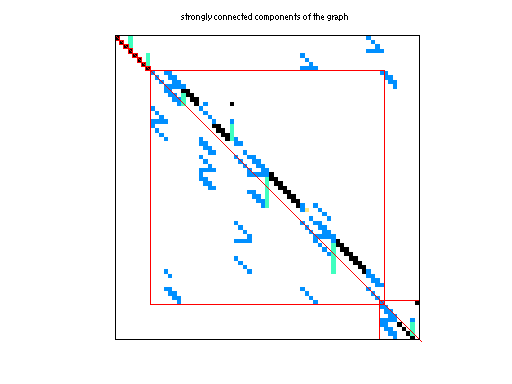Matrix: HB/impcol_c

Description: UNSYMMETRIC MATRIX - ETHYLENE PLANT MODEL (CHEM ENG) ,1982(bipartite graph drawing) (graph drawing of A+A')• Matrix group: HB
• download as a MATLAB mat-file, file size: 1 KB. Use UFget(173) or UFget('HB/impcol_c') in MATLAB.

 Matrix properties number of rows 137 number of columns 137 nonzeros 400 structural full rank? yes structural rank 137 # of blocks from dmperm 115 # strongly connected comp. 18 explicit zero entries 11 nonzero pattern symmetry 4% numeric value symmetry 1% type real structure unsymmetric Cholesky candidate? no positive definite? no

 author D. Bogle editor I. Duff, R. Grimes, J. Lewis date 1982 kind chemical process simulation problem 2D/3D problem? no

 Ordering statistics: result nnz(chol(P*(A+A'+s*I)*P')) with AMD 1,151 Cholesky flop count 1.5e+04 nnz(L+U), no partial pivoting, with AMD 2,165 nnz(V) for QR, upper bound nnz(L) for LU, with COLAMD 463 nnz(R) for QR, upper bound nnz(U) for LU, with COLAMD 1,065

Note that all matrix statistics (except nonzero pattern symmetry) exclude the 11 explicit zero entries.

 SVD-based statistics: norm(A) 120 min(svd(A)) 0.00678679 cond(A) 17681.4 rank(A) 137 sprank(A)-rank(A) 0 null space dimension 0 full numerical rank? yes

 singular values (MAT file): click here SVD method used: s = svd (full (A)) ; status: ok